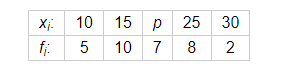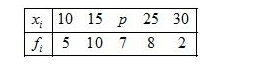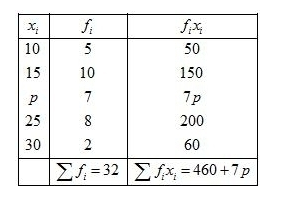# If the mean of the following data is 18.75. Find the value of p.

Question:

If the mean of the following data is 18.75. Find the value of p.Solution:

Given:Mean $=18.75$

First of all prepare the frequency table in such a way that its first column consist of the values of the variate $\left(x_{i}\right)$ and the second column the corresponding frequencies $\left(f_{i}\right)$.

Thereafter multiply the frequency of each row with corresponding values of variable to obtain third column containing $\left(f_{i} x_{i}\right)$.

Then, sum of all entries in the column second and denoted by $\sum f_{i}$ and in the third column to obtain $\sum f_{i} x_{i}$.We know that mean, $\bar{X}=\frac{\sum f_{i} x_{i}}{\sum f_{i}}$

$18.75=\frac{460+7 p}{32}$

By using cross multiplication method,

$460+7 p=600$

$7 p=600-460$

$p=\frac{140}{7}$

$=20$

Hence, $p=20$